cancel
Showing results for
Did you mean: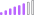Post Patron

## Create table from measures

Hi,

Is it possible to create a table out of measures?

for example I have

Mea1 = 5

Mea2 = 4

Mea3 = 1

I want to create a bar visualisation to represent these value on a chart. How can I reach that? I am thinking of putting them in a two column table, name and value.

Thanks,

1 ACCEPTED SOLUTIONCommunity Support

HI @abukapsoun,

For your scenario, you can try to use 'union' and 'row' functions.

Sample:

```Table =
UNION(ROW("Name","Measure1","Value",[Measure1]),ROW("Name","Measure2","Value",[Measure2]),ROW("Name","Measure3","Value",[Measure3]))```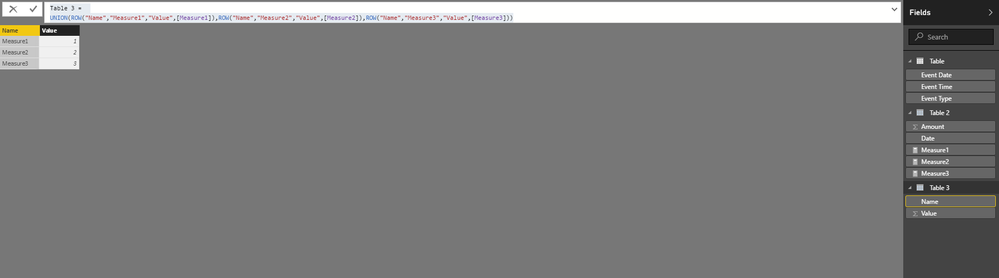Notice:

1. Above formula not suitable to create a dynamic table. if you measure values are based filter/slicer, it will show the blank as the value.

2. I haven't test on complex measures who contains specific filters and other conditions, so I'm not so sure if they can works on this formula.

3. I also test with DATATABLE function, but it seem not support dynamic value and alert me to use static value.

Regards,

Xiaoxin Sheng

Community Support Team _ Xiaoxin
If this post helps, please consider accept as solution to help other members find it more quickly.
7 REPLIES 7New Member

Hi,

how/when/where did you created the

"NewTable"

?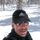Super User

Hi @Jakub_Pabisiak ,
A table can be created in Power BI or Power Query by using Enter Data. This is useful if you need to enter data, or in this case to create a table to answer a question here.Let me know if you have any questions.

If this solves your issues, please mark it as the solution, so that others can find it easily. Kudos 👍are nice too.
Nathaniel

Proud to be a Super User!Super User

Here is another post on the same subject, which I solved for a dynamic table.

Hello!

I'm trying to create a dynamic table with DAX functions where one of the columns shows the value that user input in the what-if parameter. For example if the user enter these values for what-if parameters: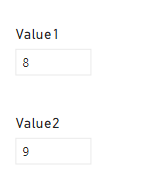My table should shows: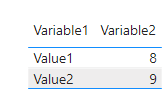I'm tryng to use this DAX function to create the table, but it don't work (the error message is: too many arguments were passed to the SELECTEDMEASURE function. The maximum argument count fot the function is 0):Any suggestion?

Thank you so much!

==================

My solution:

Try this:

• Create a table with one column with the Value names
• Create the measure below

I used an IF() but use a SWITCH () if there are more values.

Let me know if you have any questions.

If this solves your issues, please mark it as the solution, so that others can find it easily. Kudosare nice too.
Nathaniel

``````Value table =
VAR _curValue1 = 'Value 1'[Value 1 Value]
VAR _curTableValue =
MAX ( newtable[Value] )
VAR _curValue2 = 'Value 2'[Value 2 Value]
RETURN
IF ( _curTableValue = "Value 1", _curValue1, _curValue2 )``````https://1drv.ms/u/s!AgCd7AyfqZtE3z_A6wH2EyvfloHC?e=x3JuXX  This is my pbix if you can use it.

Let me know if you have any questions.

If this solves your issues, please mark it as the solution, so that others can find it easily. Kudos 👍are nice too.
Nathaniel

Proud to be a Super User!New Member

Thank you for posting this @Nathaniel_C ! If you want to add a third column would you just create a third variable and add it to the return statement? I'm having some trouble with that. Thanks!Super User

Hi @parr4 ,
Not sure what you would want to show in the third column.  If you mean add a third value then use this measure.  I commented out the if statement and added SWITCH() function.
This measure shows the values for the second column.

``````Value table =
VAR _curValue1 = 'Value 1'[Value 1 Value]
VAR _curTableValue =
MAX ( NewTable[Value] )
VAR _curValue2 = 'Value 2'[Value 2 Value]
VAR _curValue3 = 'Value 3'[Value 3 Value]
RETURN
//IF ( _curTableValue = "Value 1", _curValue1, _curValue2 )
SWITCH (
_curTableValue,
"Value 1", _curValue1,
"Value 2", _curValue2,
"Value 3", _curValue3
)
``````If you wanted to show a third column, that perhaps squares the values in the second column...

``````Square the value in column 2 =

VAR _curValue1 = 'Value 1'[Value 1 Value]
VAR _curTableValue =
MAX ( NewTable[Value] )
VAR _curValue2 = 'Value 2'[Value 2 Value]
VAR _curValue3 = 'Value 3'[Value 3 Value]
RETURN
//IF ( _curTableValue = "Value 1", _curValue1, _curValue2 )
SWITCH (
_curTableValue,
"Value 1", _curValue1 * _curValue1,
"Value 2", _curValue2 * _curValue2,
"Value 3", _curValue3 *_curValue3
)``````The first column was entered using Entered Data.  The next two columns each had their own measure.

Let me know if you have any questions.

If this solves your issues, please mark it as the solution, so that others can find it easily. Kudos 👍are nice too.
Nathaniel

Proud to be a Super User!Community Support

HI @abukapsoun,

For your scenario, you can try to use 'union' and 'row' functions.

Sample:

```Table =
UNION(ROW("Name","Measure1","Value",[Measure1]),ROW("Name","Measure2","Value",[Measure2]),ROW("Name","Measure3","Value",[Measure3]))```Notice:

1. Above formula not suitable to create a dynamic table. if you measure values are based filter/slicer, it will show the blank as the value.

2. I haven't test on complex measures who contains specific filters and other conditions, so I'm not so sure if they can works on this formula.

3. I also test with DATATABLE function, but it seem not support dynamic value and alert me to use static value.

Regards,

Xiaoxin Sheng

Community Support Team _ Xiaoxin
If this post helps, please consider accept as solution to help other members find it more quickly.Helper V

Hi @v-shex-msft,

You mean we cant use measures whose values are changing dynamically?

Thanks,

Jat